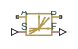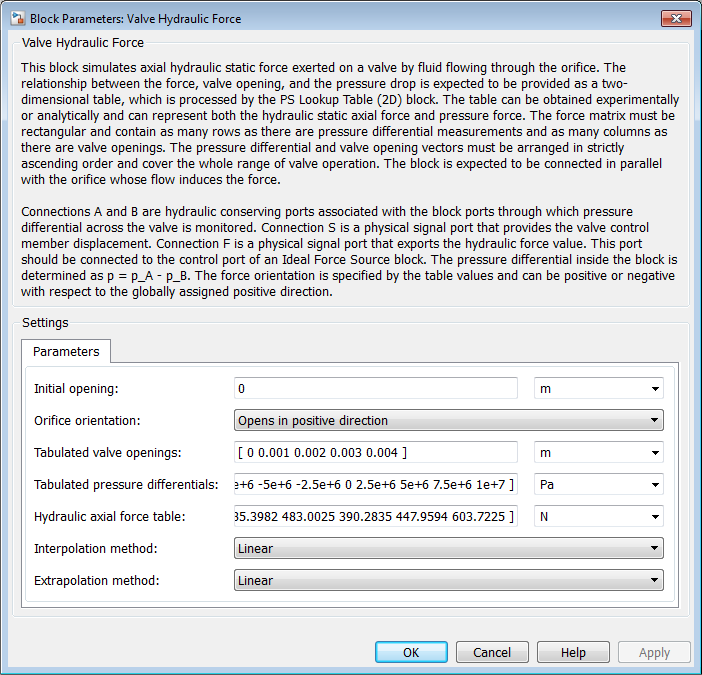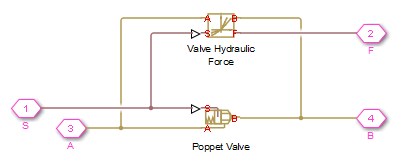# Valve Hydraulic Force

(To be removed) Axial hydraulic static force exerted on valve

The Hydraulics (Isothermal) library will be removed in a future release. Use the Isothermal Liquid library instead. (since R2020a)

## Library

Valve Forces

•## Description

The Valve Hydraulic Force block simulates axial hydraulic static force exerted on a valve by fluid flowing through the orifice. The relationship between the valve opening, the pressure drop, and the force is provided as a two-dimensional table, which is processed by the PS Lookup Table (2D) block. The table can be obtained experimentally or analytically and can represent both the hydraulic static axial force and pressure forces. The force matrix must be rectangular and contain as many rows as there are pressure differential measurements and as many columns as there are valve openings. The pressure differential and opening vectors must be arranged in strictly ascending order and cover the whole range of valve operation. Connect the block in parallel with the orifice whose flow induces the force.

Connections A and B are hydraulic conserving ports that should be connected to the valve block ports in such a way as to monitor the pressure differential across the valve. Connection S is a physical signal port that provides the valve control member displacement. Connection F is a physical signal port that outputs the hydraulic axial force value. This port should be connected to the control port of an Ideal Force Source block. The pressure differential inside the block is determined as $\Delta p={p}_{\text{A}}-{p}_{\text{B}},$. The force orientation is specified by the table values and can be positive or negative with respect to the globally assigned positive direction, depending on the value of the Orifice orientation parameter.

## Basic Assumptions and Limitations

No transient effects can be simulated.

## Dialog Box and ParametersInitial opening

Orifice initial opening. The parameter can be positive (underlapped orifice), negative (overlapped orifice), or equal to zero for zero lap configuration. The default value is `0`.

Orifice orientation

The parameter is introduced to specify the effect of the valve opening on the valve force. The parameter can be set to one of two options: ```Opens in positive direction``` or ```Opens in negative direction```. The value `Opens in positive direction` specifies an orifice that opens when the valve is shifted in the globally assigned positive direction. The default value is ```Opens in positive direction```.

Tabulated valve openings

Specify the vector of input values for valve openings as a one-dimensional array. The input values vector must be strictly increasing. The values can be nonuniformly spaced. The minimum number of values depends on the interpolation method: you must provide at least two values for linear interpolation, at least three values for smooth interpolation. The default values, in meters, are `[0,1e-3,2e-3,3e-3,4e-3]`. The Tabulated valve openings values will be used together with Tabulated pressure differentials for two-dimensional table lookup in the Hydraulic axial force table.

Tabulated pressure differentials

Specify the vector of input values for pressure differentials as a one-dimensional array. The vector must be strictly increasing. The values can be nonuniformly spaced. The minimum number of values depends on the interpolation method: you must provide at least two values for linear interpolation, at least three values for smooth interpolation. The default values, in Pa, are `[-100e5,-75e5,-50e5,-25e5,0,25e5,50e5,75e5,100e5]`.

Hydraulic axial force table

Specify the hydraulic axial force as an `m`-by-`n` matrix, where `m` is the number of valve openings and `n` is the number of pressure differentials. Each value in the matrix specifies an axial force corresponding to a specific combination of valve opening and pressure differential. The matrix size must match the dimensions defined by the input vectors. The default values, in N, are:

``` [0, -127.3576, -27.8944, 227.2513, 575.3104; ... 0, -95.5182, -20.9208, 170.4385, 431.4828; ... 0, -63.6788, -13.9472, 113.6256, 287.6552; ... 0, -31.8394, -6.9736, 56.8128, 143.8276; ... 0, 0, 0, 0, 0; ... 196.3495, 120.7506, 97.5709, 111.9898, 150.9306; ... 392.6991, 241.5013, 195.1418, 223.9797, 301.8613; ... 589.0486, 362.2519, 292.7126, 335.9695, 452.7919; ... 785.3982, 483.0025, 390.2835, 447.9594, 603.7225]```

Interpolation method

Select one of the following interpolation methods for approximating the output value when the input value is between two consecutive grid points:

• `Linear` — Select this option to get the best performance.

• `Smooth` — Select this option to produce a continuous surface with continuous first-order derivatives.

For more information on interpolation algorithms, see the PS Lookup Table (2D) block reference page.

Extrapolation method

Select one of the following extrapolation methods for determining the output value when the input value is outside the range specified in the argument list:

• `Linear` — Select this option to produce a surface with continuous first-order derivatives in the extrapolation region and at the boundary with the interpolation region.

• `Nearest` — Select this option to produce an extrapolation that does not go above the highest point in the data or below the lowest point in the data.

For more information on extrapolation algorithms, see the PS Lookup Table (2D) block reference page.

## Ports

The block has the following ports:

`A`

Hydraulic conserving port associated with a valve port.

`B`

Hydraulic conserving port associated with another valve port to monitor the pressure differential.

`S`

Physical signal port that provides the valve control member displacement.

`F`

Physical signal port that outputs hydraulic axial force.

## Examples

The following example shows a model of a poppet valve built of a Poppet Valve block and a Valve Hydraulic Force block. The Valve Hydraulic Force block is connected in parallel and provides tabulated data to compute hydraulic force acting on the valve. The force value is exported through the F port.## Version History

Introduced in R2007a

expand all